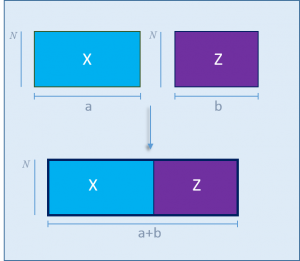# Multiblock-PLS (MB-PLS)

##MB-PLS is a multi-block regression method developed to estimate regression equations between independent blocks. Download the function plsmbExVal

This toolbox contains a MATLAB function that allow creating calibration models and then predicting unknown samples. The function implements methodology described in J.A.Westerius, T. Kourti, J.F. MacGregor, Analysis of hierarchical PCA and PLS models, J. Chemometr. 12 (1998) 301–321

The output is a structure that will contain:

Calibration Model:

• PLS parameters
• Predicted Y
• Pretreatment info

Prediction Model:

• Scores
• Predicted Y